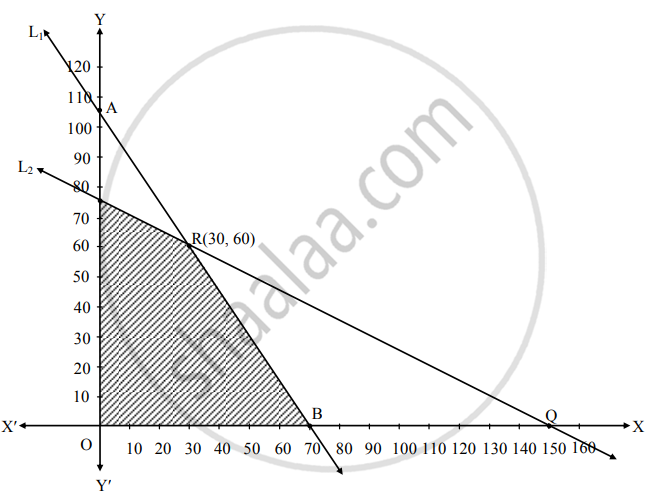# Solve the following problem : A firm manufacturing two types of electrical items A and B, can make a profit of ₹ 20 per unit of A and ₹ 30 per unit of B. Both A and B make use of two essential compo - Mathematics and Statistics

Graph
Sum

Solve the following problem :

A firm manufacturing two types of electrical items A and B, can make a profit of ₹ 20 per unit of A and ₹ 30 per unit of B. Both A and B make use of two essential components, a motor and a transformer. Each unit of A requires 3 motors and 2 transformers and each unit of B requires 2 motors and 4 transformers. The total supply of components per month is restricted to 210 motors and 300 transformers. How many units of A and B should be manufacture per month to maximize profit? How much is the maximum profit?

#### Solution

Let the firm manufacture x units of A and y units of B.
The profit is ₹ 20 per unit of A and ₹ 30 per unit of B.
∴ Total profit = ₹ (20 x + 30 y).
We construct a table with the constraints of number of motors and transformers needed.

 Electrical item\Essential component A (x) B (y) Maximum Supply Motors 3 2 210 Transformers 2 4 300

From the table, the total motors required is (3x + 2y) and total motor required is (2x + 4y).
But total supply of components per month is restricted to 210 motors and 300 transformers.
∴ The constraints are 3x + 2y ≤ 210 and 2x + 4y ≤ 300.
As x, y cannot be negative, we have x ≤ 0 and y ≥ 0.
Hence the given LPP can be formulated as follows:
Maximize Z = 20x + 30y
Subject to
3x + 2y ≤ 210,
2x + 4y ≤ 300,
x ≤ 0, y ≥ 0.
For graphical solutions of the inequalities, consider lines L1 : 3x + 2y = 210 and 2x + 4y = 300

For L1

 x y (x, y) 0 105 (0, 105) 70 0 (70, 0)

For L2

 x y (x, y) 0 75 (0, 75) 150 0 (150, 0)

L1 passes through A (0, 105) and B (70, 0)
L2 passes through P (0, 75) and Q (150, 0)
Solving both lines, we get x = 30, y = 60
The coordinates of origin O (0, 0) satisfies both the inequalities.
∴ The required region is on origin side of both the lines L1 and L2.
As x ≥ 0, y ≥ 0; the feasible region lies in the first quadrant.
OBRP is the required feasible region.
At O (0, 0), Z = 0 + 0 = 0
At B (70, 0), Z = 20 (70) + 0 = 1400
At R (30, 60), Z = 20 (30) + 30 (60) = 2400
At P (0, 75), Z = 0 + 30 (75) = 2250
The maximum value of Z is 2400 and it occurs at R (30, 60)
Thus 30 units of A and 60 units of B must be manufactured to get maximum profit of ₹ 2400.Is there an error in this question or solution?
Chapter 6: Linear Programming - Miscellaneous Exercise 6 [Page 105]

#### APPEARS IN

Balbharati Mathematics and Statistics 2 (Commerce) 12th Standard HSC Maharashtra State Board
Chapter 6 Linear Programming
Miscellaneous Exercise 6 | Q 4.15 | Page 105
Share